## Two atomic particles approach each other in a head-on collision. Each particle has a mass of 2.97 × 10-25 kg. The speed of each particle is

Question

Two atomic particles approach each other in a head-on collision. Each particle has a mass of 2.97 × 10-25 kg. The speed of each particle is 2.19 × 108 m/s when measured by an observer standing in the laboratory. (a) What is the speed of one particle as seen by the other particle? (b) Determine the relativistic momentum of one particle, as it would be observed by the other.

in progress 0
5 months 2021-09-05T11:21:13+00:00 1 Answers 0 views 0

a)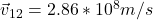b)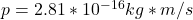Explanation:

a) When we have two particles traveling in parallel directions, the formula for relative velocity is: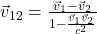Here we have that v(1) = -v(2), the speed of the of the second particle is the negative of the first one.

If we use these equivalence we have: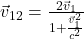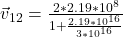And,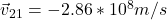b) The relativistic momentum equation to one particle observed by the other particle, is: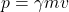Where gamma is: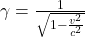• v is the speed of the first particle relative to the second particle (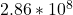)
• m is the mass of the particle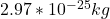Then gamma will be: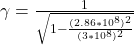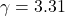Finally, the value of the momentum will be: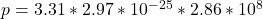I hope it helps you!